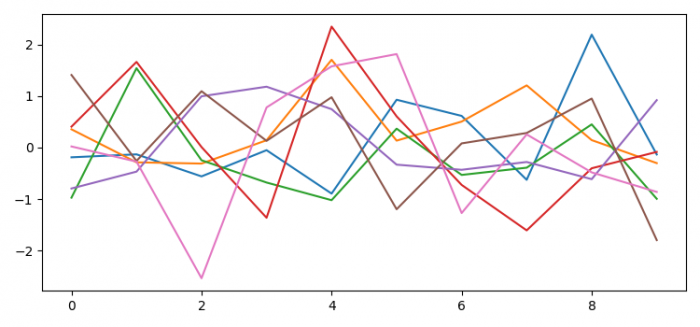# How to manipulate figures while a script is running in Python Matplotlib?

To manipulate figures while a script is running in Python, we can take the following steps −

• Set the figure size and adjust the padding between and around the subplots.
• Create a new figure or activate an existing figure using figure() method.
• Get the current axis, ax, and show the current figure.
• Manipulate the script using plt.pause() method, before the final plot.
• Plot the line using plot() method.
• To display the figure, use show() method.

## Example

import numpy as np
from matplotlib import pyplot as plt

plt.rcParams["figure.figsize"] = [7.50, 3.50]
plt.rcParams["figure.autolayout"] = True

fig = plt.figure()
ax = fig.gca()
fig.show()

for i in range(20):
ax.plot(np.random.randn(10, 1), ls='-')
fig.canvas.draw()
plt.pause(0.1)

plt.close(fig)
plt.plot([1, -2, 3, 5, 3, 1, 0])

plt.show()

## Output